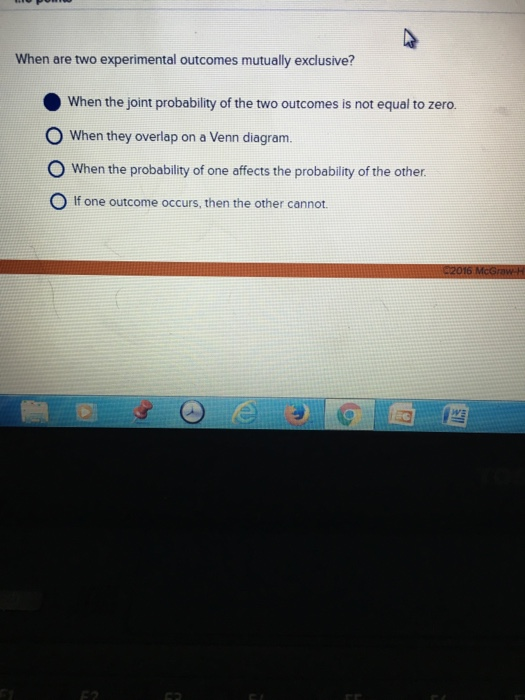# When are two experimental outcomes mutually exclusive?When are two experimental outcomes mutually exclusive? When the joint probability of the two outcomes is not equal to zero. When they overlap on a Venn diagram. When the probability of one affects the probability of the other. If one outcome occurs, then the other cannot.

d. if the one outcome occurs, other can not